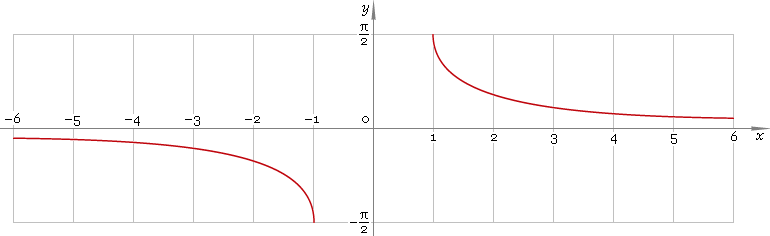The Art of Interface

# arccsc or arccosec — trigonometric arc cosecant function

Category. Mathematics.

Abstract. Trigonometric arc cosecant: definition, plot, properties, identities and table of values for some arguments.

## 1. Definition

Arc cosecant is inverse of the cosecant function.

## 2. Plot

Arc cosecant is antisymmetric function defined everywhere on real axis, ecxept the range (−1, 1) — so, function domain is (−∞, −1]∪[1, +∞). Its plot is depicted below — fig. 1Fig. 1. Plot of the arc cosecant function y = arccscx.

Function codomain is limited to the range [−π/2, 0)∪(0, π/2].

## 3. Identities

Complementary angle:

arcsecx + arccscx = π/2

and as consequence:

arccsc sec φ = π/2 − φ

Negative argument:

arccsc(−x) = −arccscx

Reciprocal argument:

arcsc(1/x) = arcsinx

Sum and difference:

arccscx + arccscy = arccsc{xy / [x√(1 − 1 /x2) + y√(1 − 1 /y2)]}
arccscx − arccscy = arccsc{xy / [y√(1 − 1 /y2) − x√(1 − 1 /x2)]}

Some argument values:

Argument xValue arccscx
1π/2
√6 − √25π/12
√(50 − 10√5) /52π/5
√(2 − √2)3π/8
2√3 /3π/3
√5 − 13π/10
√2π/4
√(50 + 10√5) /5π/5
2π/6
√(4 + 2√2)π/8
√5 + 1π/10
√6 + √2π/12
Table 1. Arc cosecant for some argument values.

## 4. Support

Trigonometric arc cosecant function arccsc or arccosec of the real argument is supported by free version of the Librow calculator.

Trigonometric arc cosecant function arccsc or arccosec of the complex argument is supported by professional version of the Librow calculator.

## 5. How to use

To calculate arc cosecant of the number:

``arccsc(-1);``

To calculate arc cosecant of the current result:

``arccsc(rslt);``

To calculate arc cosecant of the number x in memory:

``arccsc(mem[x]);``